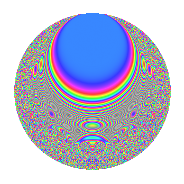# Properties

 Label 525.4.gLevel $525$ Weight $4$ Character orbit 525.g Rep. character $\chi_{525}(524,\cdot)$ Character field $\Q$ Dimension $140$ Sturm bound $320$

# Related objects

## Defining parameters

 Level: $$N$$ $$=$$ $$525 = 3 \cdot 5^{2} \cdot 7$$ Weight: $$k$$ $$=$$ $$4$$ Character orbit: $$[\chi]$$ $$=$$ 525.g (of order $$2$$ and degree $$1$$) Character conductor: $$\operatorname{cond}(\chi)$$ $$=$$ $$105$$ Character field: $$\Q$$ Sturm bound: $$320$$

## Dimensions

The following table gives the dimensions of various subspaces of $$M_{4}(525, [\chi])$$.

Total New Old
Modular forms 252 148 104
Cusp forms 228 140 88
Eisenstein series 24 8 16

## Trace form

 $$140q + 552q^{4} + 92q^{9} + O(q^{10})$$ $$140q + 552q^{4} + 92q^{9} + 2104q^{16} + 60q^{21} + 1176q^{36} + 384q^{39} + 2480q^{46} - 32q^{49} - 1968q^{51} + 11584q^{64} - 3376q^{79} - 4820q^{81} + 1044q^{84} - 1116q^{91} - 8696q^{99} + O(q^{100})$$

## Decomposition of $$S_{4}^{\mathrm{new}}(525, [\chi])$$ into newform subspaces

The newforms in this space have not yet been added to the LMFDB.

## Decomposition of $$S_{4}^{\mathrm{old}}(525, [\chi])$$ into lower level spaces

$$S_{4}^{\mathrm{old}}(525, [\chi]) \cong$$ $$S_{4}^{\mathrm{new}}(105, [\chi])$$$$^{\oplus 2}$$

## Hecke characteristic polynomials

There are no characteristic polynomials of Hecke operators in the database